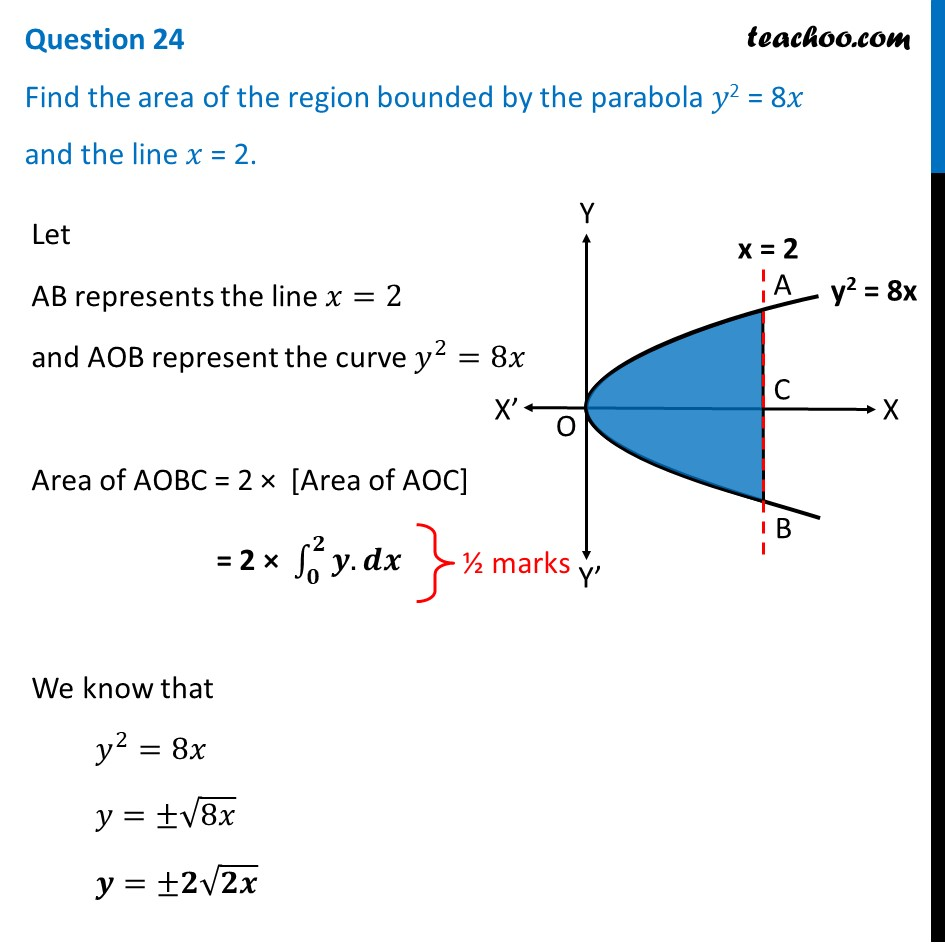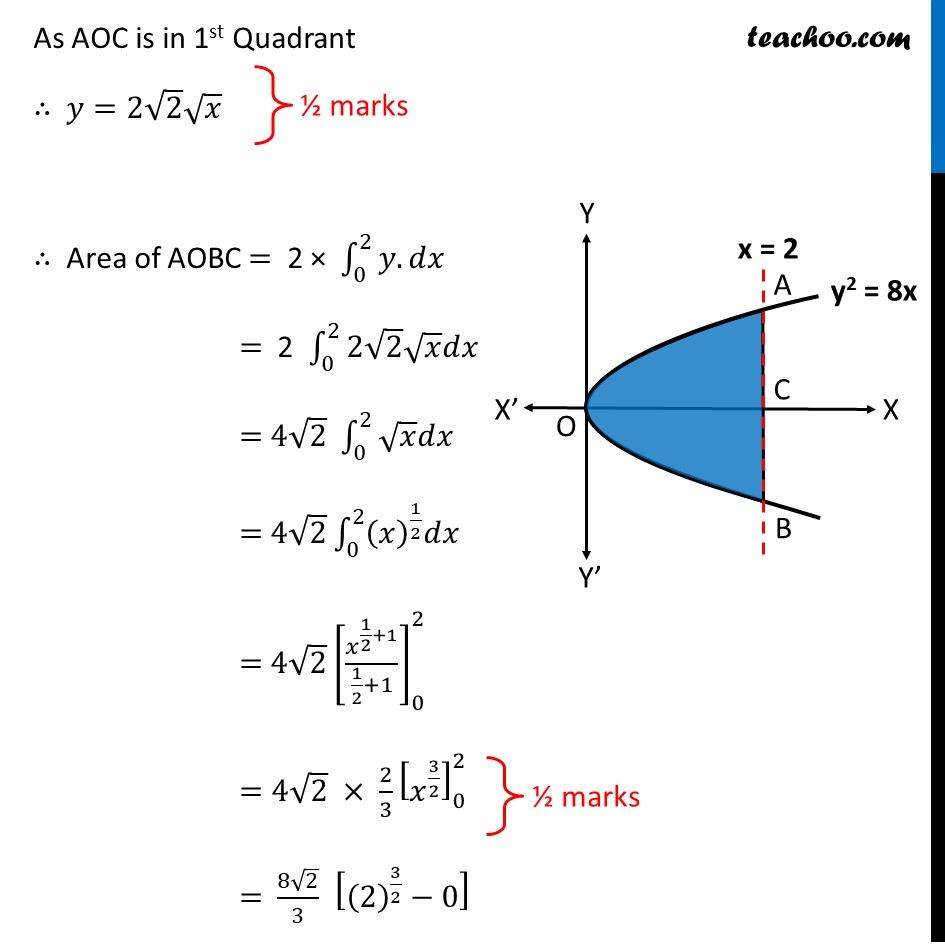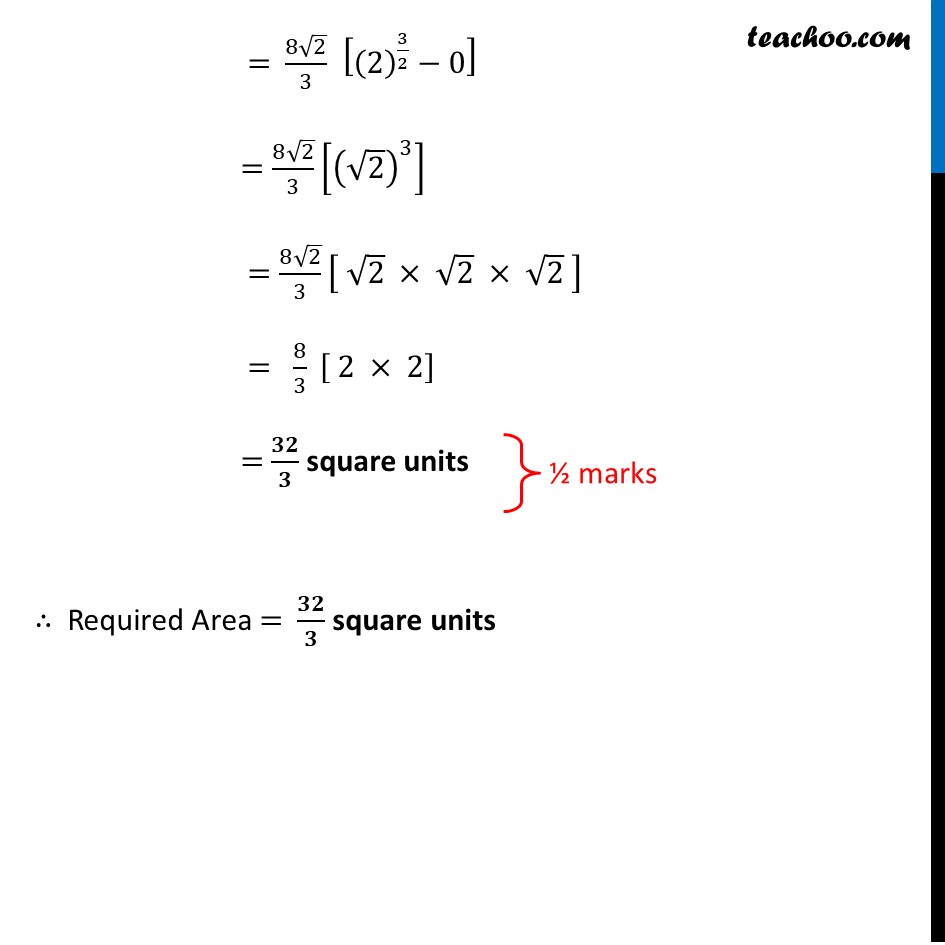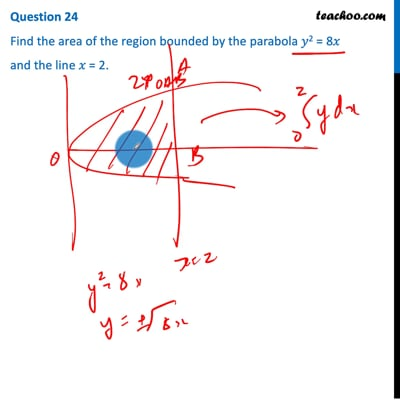CBSE Class 12 Sample Paper for 2021 Boards

Class 12
Solutions of Sample Papers and Past Year Papers - for Class 12 Boards

## Find the area of the region bounded by the parabola 𝑦 2 = 8𝑥 and the line 𝑥 = 2.This video is only available for Teachoo black users

Note : This is similar to Ex 8.1, 11 of NCERT – Chapter 8 Class 12 Applications of Integration

Check the answer here https:// www.teachoo.com /3335/730/Ex-8.1--11---Find-area-bounded-by-y2--4x-and-line-x--3/category/Ex-8.1/

Introducing your new favourite teacher - Teachoo Black, at only ₹83 per month

### Transcript

Question 24 Find the area of the region bounded by the parabola 𝑦2 = 8𝑥 and the line 𝑥 = 2. Let AB represents the line 𝑥=2 and AOB represent the curve 𝑦^2=8𝑥 Area of AOBC = 2 × [Area of AOC] = 2 × ∫_𝟎^𝟐▒〖𝒚.𝒅𝒙〗 We know that 𝑦^2=8𝑥 𝑦=±√8𝑥 𝒚=±𝟐√𝟐𝒙 As AOC is in 1st Quadrant ∴ 𝑦=2√2 √𝑥 ∴ Area of AOBC = 2 × ∫_0^2▒〖𝑦.𝑑𝑥〗 = 2 ∫_0^2▒〖2√2 √𝑥 𝑑𝑥〗 = 4√2 ∫_0^2▒〖√𝑥 𝑑𝑥〗 = 4√2 ∫_0^2▒〖(𝑥)^(1/2) 𝑑𝑥〗 = 4√2 [𝑥^(1/2+1)/(1/2+1)]_0^2 =4√2 × 2/3 [𝑥^(3/2) ]_0^2 = (8√2)/3 [(2)^(3/2)−0] = (8√2)/3 [(2)^(3/2)−0] =(8√2)/3 [(√2)^3 ] =(8√2)/3 [ √2 × √2 × √2 ] = 8/3 [ 2 × 2] = 𝟑𝟐/𝟑 square units ∴ Required Area = 𝟑𝟐/𝟑 square units